# Algebra 1 : How to find consecutive integers

## Example Questions

### Example Question #2 : Quadratic Formula

The product of two consective positive odd integers is 143. Find both integers.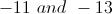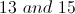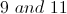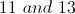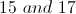Explanation:

Ifis one odd number, then the next odd number is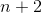. If their product is 143, then the following equation is true.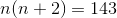Distribute into the parenthesis.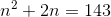Subtract 143 from both sides.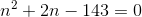This can be solved by factoring, or by the quadratic equation. We will use the latter.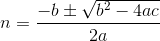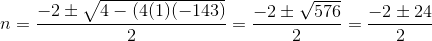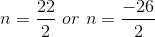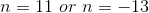We are told that both integers are positive, so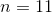.

The other integer is.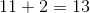### Example Question #1 : How To Find Consecutive Integers

Write a rule for the following arithmetic sequence: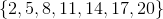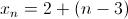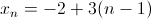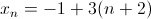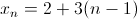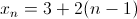Explanation:

Know that the general rule for an arithmetic sequence is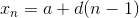,

whererepresents the first number in the sequence,is the common difference between consecutive numbers, andis the-th number in the sequence.

In our problem,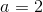.

Each time we move up from one number to the next, the sequence increases by 3.  Therefore,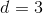The rule for this sequence is therefore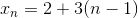.

### Example Question #2 : How To Find Consecutive Integers

If the rule of some particular sequence is written as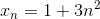,

find the first five terms of this sequence

none of these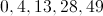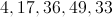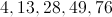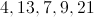Explanation:

The first term for the sequence is where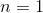. Thus,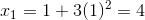So the first term is 4.  Repeat the same thing for the second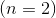, third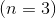, fourth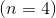, and fifth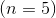terms.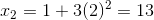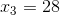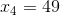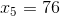We see that the first five terms in the sequence are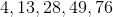### Example Question #3 : How To Find Consecutive Integers

What are three consecutive numbers that are equal to?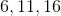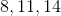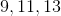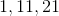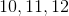Explanation:

When finding consecutive numbers assign the first number a variable.

If the first number is assigned the letter n, then the second number that is consecutive must be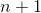and the third number must be.

Write it out as an equation and it should look like: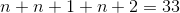Simplify the equation then,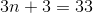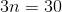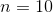Ifthen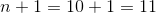And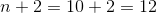So the answer is### Example Question #4 : How To Find Consecutive Integers

The sum of five odd consecutive numbers add to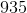. What is the fourth largest number?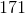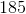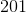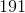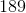Explanation:

Let the first number be.

Ifis an odd number, the next odd numbers will be: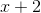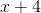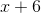, and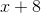The fourth highest number would then be:Set up an equation where the sum of all these numbers add up to.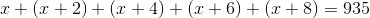Simplify this equation.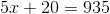Subtract 20 from both sides.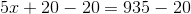Simplify both sides.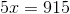Divide by five on both sides.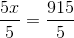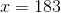Corresponding to the five numbers, the set of five consecutive numbers that add up toare: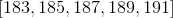The fourth largest number would be.